# Fraction calculator

The calculator performs basic and advanced operations with fractions, expressions with fractions combined with integers, decimals, and mixed numbers. It also shows detailed step-by-step information about the fraction calculation procedure. Solve problems with two, three, or more fractions and numbers in one expression.

## Result:

### 2 : (4/5) = 5/2 = 2 1/2 = 2.5

Spelled result in words is five halfs (or two and one half).

### How do you solve fractions step by step?

1. Divide: 2 : 4/5 = 2/1 · 5/4 = 2 · 5/1 · 4 = 10/4 = 2 · 5 /2 · 2 = 5/2
Dividing two fractions is the same as multiplying the first fraction by the reciprocal value of the second fraction. The first sub-step is to find the reciprocal (reverse the numerator and denominator, reciprocal of 4/5 is 5/4) of the second fraction. Next, multiply the two numerators. Then, multiply the two denominators. In the next intermediate step, , cancel by a common factor of 2 gives 5/2.
In words - two divided by four fifths = five halfs.

#### Rules for expressions with fractions:

Fractions - use the slash “/” between the numerator and denominator, i.e., for five-hundredths, enter 5/100. If you are using mixed numbers, be sure to leave a single space between the whole and fraction part.
The slash separates the numerator (number above a fraction line) and denominator (number below).

Mixed numerals (mixed fractions or mixed numbers) write as non-zero integer separated by one space and fraction i.e., 1 2/3 (having the same sign). An example of a negative mixed fraction: -5 1/2.
Because slash is both signs for fraction line and division, we recommended use colon (:) as the operator of division fractions i.e., 1/2 : 3.

Decimals (decimal numbers) enter with a decimal point . and they are automatically converted to fractions - i.e. 1.45.

The colon : and slash / is the symbol of division. Can be used to divide mixed numbers 1 2/3 : 4 3/8 or can be used for write complex fractions i.e. 1/2 : 1/3.
An asterisk * or × is the symbol for multiplication.
Plus + is addition, minus sign - is subtraction and ()[] is mathematical parentheses.
The exponentiation/power symbol is ^ - for example: (7/8-4/5)^2 = (7/8-4/5)2

#### Examples:

subtracting fractions: 2/3 - 1/2
multiplying fractions: 7/8 * 3/9
dividing Fractions: 1/2 : 3/4
exponentiation of fraction: 3/5^3
fractional exponents: 16 ^ 1/2
adding fractions and mixed numbers: 8/5 + 6 2/7
dividing integer and fraction: 5 ÷ 1/2
complex fractions: 5/8 : 2 2/3
decimal to fraction: 0.625
Fraction to Decimal: 1/4
Fraction to Percent: 1/8 %
comparing fractions: 1/4 2/3
multiplying a fraction by a whole number: 6 * 3/4
square root of a fraction: sqrt(1/16)
reducing or simplifying the fraction (simplification) - dividing the numerator and denominator of a fraction by the same non-zero number - equivalent fraction: 4/22
expression with brackets: 1/3 * (1/2 - 3 3/8)
compound fraction: 3/4 of 5/7
fractions multiple: 2/3 of 3/5
divide to find the quotient: 3/5 ÷ 2/3

The calculator follows well-known rules for order of operations. The most common mnemonics for remembering this order of operations are:
PEMDAS - Parentheses, Exponents, Multiplication, Division, Addition, Subtraction.
BEDMAS - Brackets, Exponents, Division, Multiplication, Addition, Subtraction
BODMAS - Brackets, Of or Order, Division, Multiplication, Addition, Subtraction.
GEMDAS - Grouping Symbols - brackets (){}, Exponents, Multiplication, Division, Addition, Subtraction.
Be careful, always do multiplication and division before addition and subtraction. Some operators (+ and -) and (* and /) has the same priority and then must evaluate from left to right.

## Fractions in word problems:

• Divide 13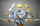Divide. Simplify your answer and write as an improper fraction or whole number. 14÷8/3
• Product of two fractionsProduct of two fractions is 9 3/5 . If one of the fraction is 9 3/7. Find the other fraction.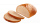There are 12 slices of bread and each person gets 3/4 of a slice of bread. How many people get bread?​
• A shopkeeper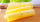A shopkeeper cuts a wheel of cheese into 10 equal wedges. A customer buys one-fifth of the wheel. How many wedges does the customer buy? Use the number line to help find the solution.
• Unit rateFind unit rate: 6,840 customers in 45 days
• Mixed divided with fracThere are 2 2/3 pizzas. How many people are sharing when each has 2/3 of pizza?
• Soup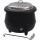On Monday we cook 25 pots and 10 boilers of soup. On Tuesday 15 pots and 13 boilers. On Wednesday 20 pots and on Thursday 30 boilers. On Monday and Tuesday was cooked the same amount of soup. How many times more soup cooked on Thursday than on Wednesday?
• Reciprocal value 2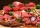Annabelle is making burger patties if each burger patty needs 1/16 kilogram of beef. How many patties can she make with a kilo of beef?
• ExpressionsLet k represent an unknown number, express the following expressions: 1. The sum of the number n and two 2. The quotient of the numbers n and nine 3. Twice the number n 4. The difference between nine and the number n 5. Nine less than the number n
• Unknown number4/5 of a number is 276. what is 2/3 of the same number?
• 6/24 change6/24 change to a fraction in lowest terms
• Mineral water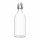The bottle contains 1.5 liters of mineral water. Pour all the water from the bottle into empty cups with a volume of 1/3 l. All but one will be filled to the brim. What part of the volume of the last cup is filled with water?
• James 2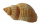James collected 24 seashells on the seashore to be shared with his 2 friends. What fractional part of the seashells will each one get if it is distributed evenly? ​